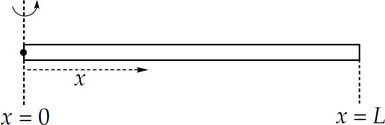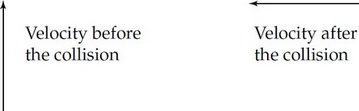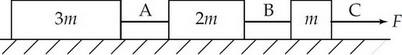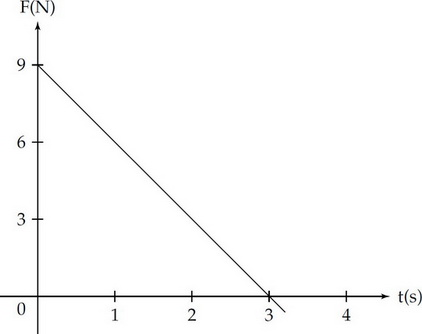# AP Physics C: Mechanics Practice Test 4

### Test Information10 questions13 minutes

1.The rod shown above can pivot about the point x = 0 and rotates in a plane perpendicular to the page. Its linear density, λ, increases with x such that λ(x) = kx, where k is a positive constant. Determine the rod's moment of inertia in terms of its length, L, and its total mass, M.

2. A particle is subjected to a conservative force whose potential energy function is

U(x) = (x – 2)3 – 12x

where U is given in joules when x is measured in meters. Which of the following represents a position of stable equilibrium?

3. At what angle to the horizontal should an ideal projectile be launched so that its horizontal displacement (the range) is equal to its maximum vertical displacement?

4. A particle's kinetic energy is changing at a rate of –6.0 J/s when its speed is 3.0 m/s. What is the magnitude of the force on the particle at this moment?

5. An object of mass 2 kg is acted upon by three external forces, each of magnitude 4 N. Which of the following could NOT be the resulting acceleration of the object?

6.The graph above shows the velocity vs. time graph for a 3 kg object moving in one dimension. Which of the following is a possible graph of position versus time for this object?

7. A ball is dropped from an 80 m tall building. How long does the ball take to reach the ground?

8.The velocity of an object before a collision is directed straight north and the velocity after the collision is directed straight west, as shown above. Which of the following vectors represents the change in momentum of the object?

9.Three blocks of masses 3m, 2m, and m are connected to strings A, B, and C as shown above. The blocks are pulled along a frictionless, horizontal floor with a force, F. Determine the acceleration of the 2m block.

10.A block of mass 2 kg, initially at rest, is pulled along a frictionless, horizontal surface with a force shown as a function of time by the graph above.

The acceleration of the block at t = 2 s is